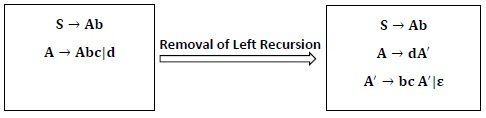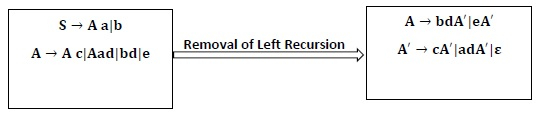# What is Non-Immediate Left Recursion in compiler design?

A Grammar G (V, T, P, S) is left recursive if it has a production in the form.

A → A α |β.

The above Grammar is left recursive because the left of production is occurring at a first position on the right side of production. It can eliminate left recursion by replacing a pair of production with

A → βA′

A → αA′|Ďµ

The general form for left recursion is

A → Aα1|Aα2| … . |Aαm12| … . . βn

can be replaced by

A → β1A′|β2A′| … . . | … . . |βnA′

A → α1A′|α2A′| … . . |αmA′|ε

In the following grammar, it does not have the immediate or direct left recursion. But here, it can have non-immediate left recursion.

S → Ab

A → Sc|d

Here, S is left Recursive, because S ⇒ A b ⇒ S c b

Algorithm to Remove Left-Recursion

Input − Grammar G with no cycles or ε − productions.

Output − Equivalent Grammar with no Left Recursion.

Method

• Order the non-terminals as A1, A2, … … … . . An

for i = 1 to n
{
for j = 1 to i – 1
{
replace each production Ai → Ajγ
by productions Ai → δ1γ| δ2γ| … … . . |δKγ
where Aj → δ1|δ2| … … . |δK
}
Remove immediate left Recursion among Ai productions.
}

Example1 − Remove or Eliminate Left Recursion from the following Grammar.

S → Ab

A → Sc | d

Solution

These productions don’t have immediate left Recursion. So it cannot be eliminated directly. We can use the algorithm here to remove Left Recursion.

Step1 − Order the non-terminals S, A as A1, A2i.e., Mark S =A1, A = A2

Substituting values

∴   A1 → A2b                             …………… (1)

A2 → A1c|d                           …………… (2)

Step2 − Put values of (1) in (2)

∴  A1 → A2b

A2 → A1bc|d

Step3 − Again substitutes A1 = S and A2 = A

∴  S → A b                              ……………. (3)

A → A b c | d                        ……………. (4)

Step4 − Remove Immediate Left recursion in A → A b c | d

So,Example2 − Remove left recursion from the following Grammar.

S → Aa |b

A → Ac | Sd|e

Solution

As we don’t have immediate left recursion so, we have to apply the algorithm here.

Step1 − Rename S, A as A1, A2 respectively.

A1 → A2 a | b                        …………… (1)

A2 → A2c | A1d|e                  ……………. (2)

Step2 − Substitute value of A1 of the statement (1) in R.H.S of the statement (2).

A1 → A2 a | b

A2 → A2 c |A2 a d|bd|e.

Step3 − Again, substitute A1 = S, A2 = A

S → Aa |b                               …………….. (3)

A → Ac |A a d|bd|e                   ……………… (4)

Step4 − Statement (4) has an immediate left recursion. Removing immediate left recursion we get